## Example Questions

### Example Question #11 : Inequalities

(√(8) / -x ) <  2. Which of the following values could be x?

-4

-3

All of the answers choices are valid.

-2

-1

-1

Explanation:

The equation simplifies to x > -1.41. -1 is the answer.

### Example Question #51 : New Sat Math Calculator

Solve for x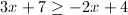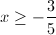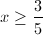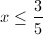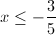Explanation: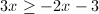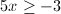### Example Question #5 : How To Find The Solution To An Inequality With Multiplication

We have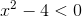, find the solution set for this inequality.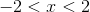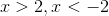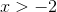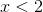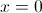Explanation: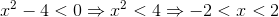### Example Question #1 : How To Find The Solution To An Inequality With Multiplication

Fill in the circle with either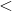,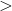, orsymbols: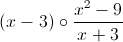for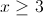.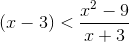The rational expression is undefined.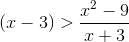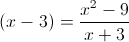None of the other answers are correct.Explanation:Let us simplify the second expression. We know that: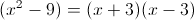So we can cancel out as follows: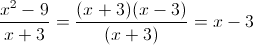### Example Question #1 : How To Find The Solution To An Inequality With Multiplication

Solve the inequality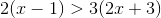.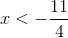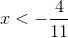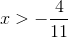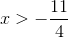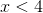Explanation:

Start by simplifying the expression by distributing through the parentheses to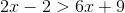.

Subtractfrom both sides to get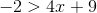.

Next subtract 9 from both sides to get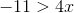. Then divide by 4 to get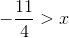which is the same as.

### Example Question #1 : How To Find The Solution To An Inequality With Multiplication

Solve the inequality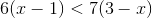.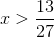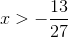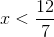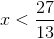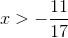Explanation:

Start by simplifying each side of the inequality by distributing through the parentheses.

This gives us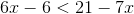.

Add 6 to both sides to get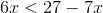.

Add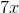to both sides to get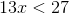.

Divide both sides by 13 to get.

### Example Question #1 : How To Find The Solution To An Inequality With Addition

Quantitative Comparison

Quantity A: 3x + 4y

Quantity B: 4x + 3y

The relationship cannot be determined from the information given.

The two quantities are equal.

Quantity A is greater.

Quantity B is greater.

The relationship cannot be determined from the information given.

Explanation:

The question does not give us any specifics about the variables x and y.

If we substitute the same numbers for x and y (say, x = 1 and y = 1), the two expressions are equal.

If we substitute different number in for x and y (say, x = 2 and y = 1), the two expressions are not equal.

If there are two possible outcomes, then we need more information to determine which quantity is greater. Don't be afraid to pick "The relationship cannot be determined from the information given" as an answer choice on the GRE!

### Example Question #1 : How To Find The Solution To An Inequality With Addition

Letbe an integer such that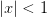.

Quantity A: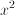Quantity B: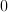The relationship cannot be determined from the information given.

Quantity A and Quantity B are equal.

Quantity A is greater.

Quantity B is greater.

Quantity A and Quantity B are equal.

Explanation:

The expressioncan be rewritten as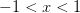.

The only integer that satisfies the inequality is 0.

Thus, Quantity A and Quantity B are equal.

### Example Question #181 : Equations / Inequalities

Find all solutions of the inequality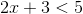.

All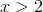.

All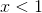..

All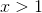.

All.

All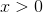.

All..

Explanation:

Start by subtracting 3 from each side of the inequality. That gives us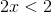. Divide both sides by 2 to get. Therefore every value forwhereis a solution to the original inequality.

### Example Question #21 : Inequalities

Find all solutions of the inequality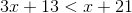.

All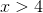.

All.

All.

All.

All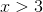.

All.
Start by subtracting 13 from each side. This gives us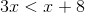. Then subtractfrom each side. This gives us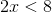. Divide both sides by 2 to get. Therefore all values ofwherewill satisfy the original inequality.